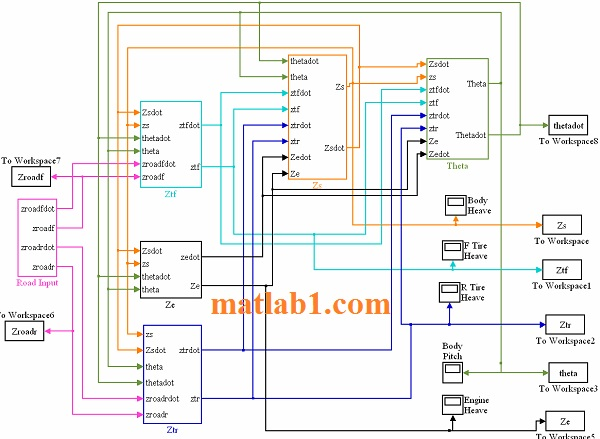Sale!

# Simulink model of a BMW Mini Cooper using the vehicle model

15

## Description

MATLAB and Simulink were used to create a ride simulation model of a BMW Mini Cooper using the vehicle model.

The simulation was used to determine the stiffness and damping parameters of the TWEEL that would yield a vehicle that had a more comfortable ride than that of the vehicle equipped with the OE tire. The simulations were done in the time domain. This allowed non-symmetric, non-linear components to be modeled.

The vehicle ride simulation was programmed using MATLAB and Simulink. Simulink is a program that allows the user to model, simulate, and analyze dynamic systems. Block diagrams are utilized to build the equations of motion. The top-level block diagram is shown in Figure 1. In the block diagram schematic, Figure 1, each of the labeled boxes, Zs, Ztf, Ztr, Ze, and Theta are the building blocks for the equation of motion for the chassis vertical motion, the front tire vertical motion, the rear tire vertical motion, the engine vertical motion, and the chassis pitch motion respectively.